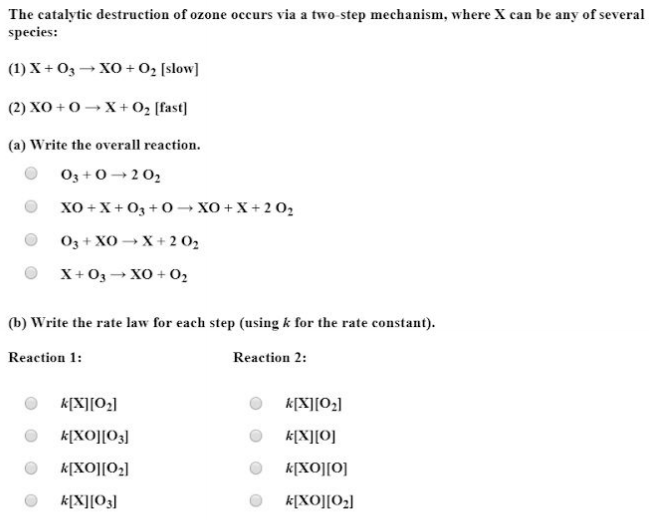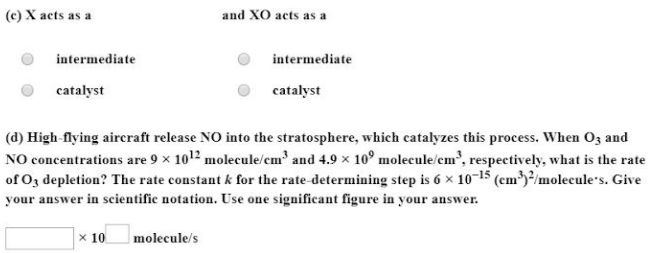# The catalytic destruction of ozone occurs via a two-step mechanism, where X can be any of several species: (1) X + O3 -> XO + O2 [slow] (2) XO + O -> X + O2 [fast] (a) Write the overall reaction. (b) Write the rate law for each step (using k for the rate constant). (c) X acts as a ___ and XO acts as a ___ (d) High-flying aircraft release NO into the stratosphere, which catalyzes this process. When O3 and NO concentrations are 9 x 10^12 molecule/cm^3 and 4.9 x 10^9 molecule/cm^3, respectively, what is the rate of O3 depletion? The rate constant k for the rate-determining step is 6 x 10^-15(cm^3)^2/molecule*s. Give your answer in scientific notation. Use one significant figure on your answer.